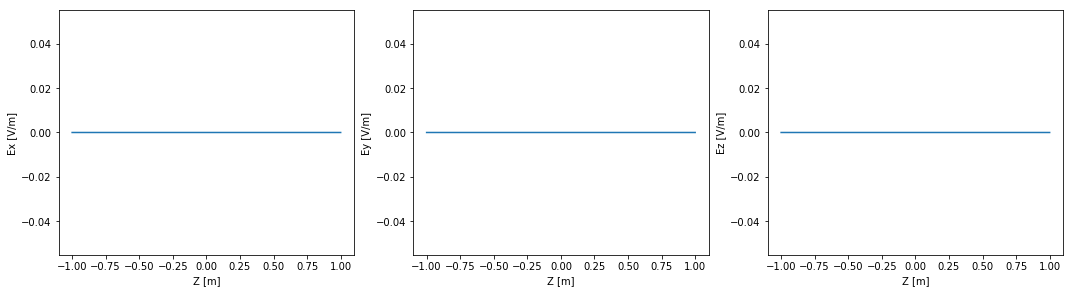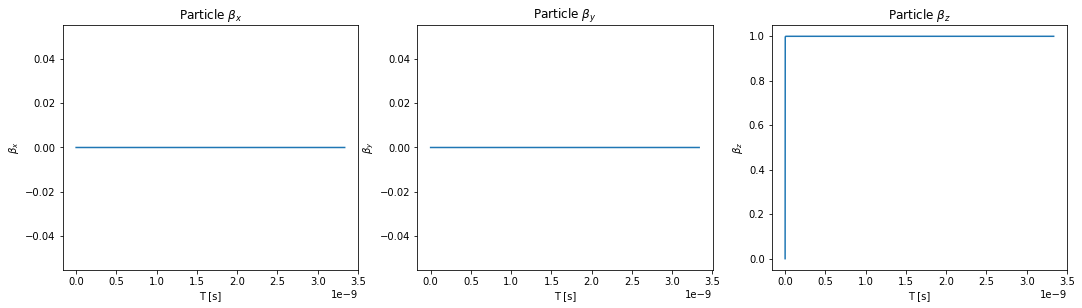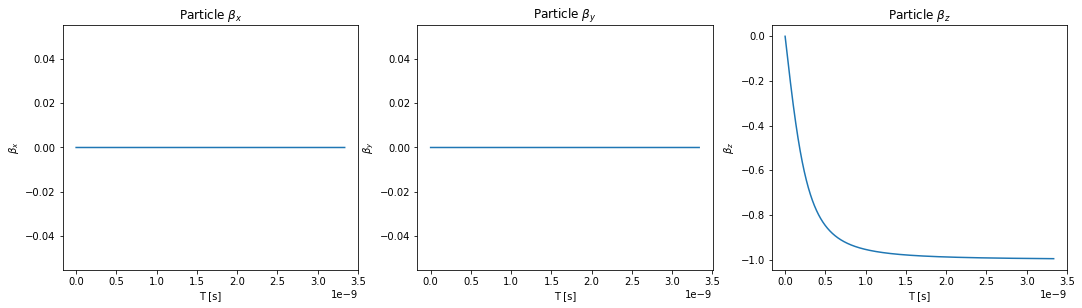# Electric Fields - All About¶

The functions available for electric fields are identical as for magnetic fields, but with the 'bfield' keyword replaced by 'efield'.

In :
# matplotlib plots inline
%matplotlib inline

# Import the OSCARS SR module
import oscars.sr

# Import OSCARS plots (matplotlib)
from oscars.plots_mpl import *

OSCARS v2.1.8 - Open Source Code for Advanced Radiation Simulation
Brookhaven National Laboratory, Upton NY, USA
http://oscars.bnl.gov
oscars@bnl.gov

In :
# Create a new OSCARS SR object
osr = oscars.sr.sr()


## Strong Field¶

Let's start with a strong field for acceleration making sure the stepsize is small enough.

In :
# Clear any previous fields and add a constant large field
# in the negative Z-direction
osr.clear_efields()

# Plot the field if you like
plot_efield(osr)In :
# Setup an electron beam with zero energy at X=Y=Z=0 (These are all defaults so no arguments given)
osr.set_particle_beam()
osr.set_ctstartstop(0, 1)

In :
# Calculate trajectory
trajectory = osr.calculate_trajectory()
plot_trajectory_velocity(trajectory)## Proton¶

Other particle types are also possible, for instance proton. For a full list see the all about particle beams.

In :
# Setup a proton beam with zero energy at Z=0
osr.set_particle_beam(type='proton')
osr.set_ctstartstop(0, 1)

In :
# Calculate trajectory
trajectory = osr.calculate_trajectory()
plot_trajectory_velocity(trajectory)